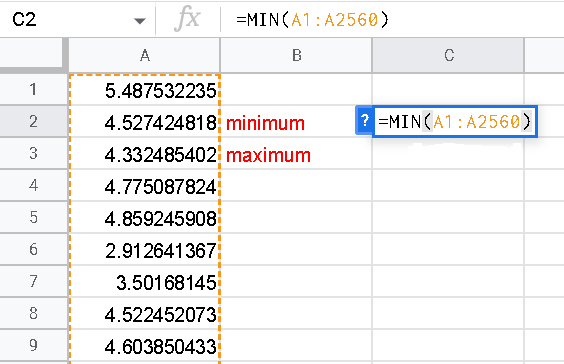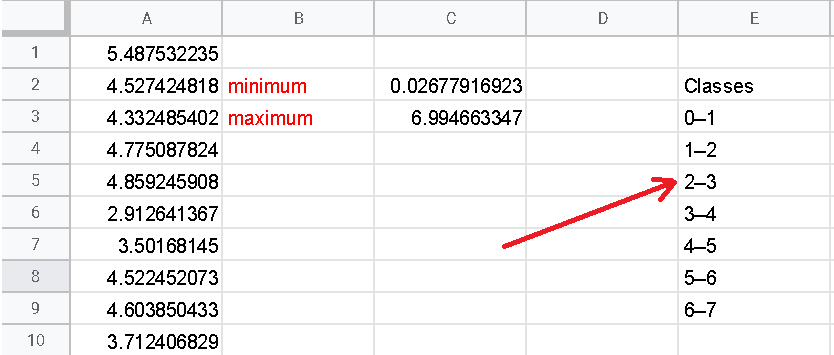# Frequency Distribution and Histogram Using Google Sheets

The steps to group data into classes, determine the frequencies of all classes and make a histograms using Google Sheets are presented.
The data used, is included in a Google sheet data file (1) and may be downloaded and used for practice.

Step 1 - Organize the data values in a single column as shown below. Only the first 20 rows are shown, but there is a total of 2560 data values included in the file Google sheet data file (1) used to make the histogram. The data values are located in the cells A1 to A2560.Step 2 - Use Google sheets "MIN" function to find the minimum of the data values in the cells A1 to A2560.
Type the functions "=MIN(A1:A2560)" and press "Enter".Step 3 - Use Google sheets "MAX" function to find the maximum of the data values in the cells A1 to A2560.
Type the functions "=MAX(A1:A2560)" and press "Enter".2) The results should be as followsStep 4 - We start by using a class width equal to 1 and calculate $N$ the number of classes and their limits. The minimum (rounded), the maximum (rounded) and the class width determined above are used to determine $N$ as follows:
$N = \dfrac{\text{Maximum - Minimum} }{\text{Class width}} = \dfrac{6.994 - 0.027}{1} = 6.967$

Round the number of classes $N$ to the nearest integer $7$.
Start from the nearest "easy to read" value close to the minimum. The minimum (rounded) of this data is $0.027$ and it makes sense to start the classes from 0 in order to make them easy to read when we interpret the histogram.
Write the limits of the classes starting from 0 adding the class width which (abbreviated as CW) is equal to 1:
The lower limit of the first class: 0
Upper limit of the first class: 0 + CW = 0 + 1 = 1
So the first class is: [0 , 1]
Second class is: [0 + CW , 1 + CW] = [1 , 2]
Third class is: [1 + CW , 2 + CW] = [2 , 3]
Fourth class is: [2 + CW , 3 + CW] = [3 , 4]
Fifth class is: [3 + CW , 4 + CW] = [4 , 5]
Sixth class is: [4 + CW , 5 + CW] = [5 , 6]
Seventh class is: [5 + CW , 6 + CW] = [6 , 7]
We stop at the upper limit 7 since the maximum data value is 6.994 and we, therefore, cover all the data values in the set with these classes.

Step 5 - Use the class limits calculated above to write the classes as shown below.Step 6 - Define the bins to be used in order to determine the frequency of each class. The Bins are the upper limit of each class.Step 7 - Use the "FREQUENCY" function in Goole sheets to determine the frequencies by selecting an empty cell (G3 in this example) beside the Bins and type "=FREQUENCY(A1:A2560,F3:F9).
NOTE that the cells A1:A2560 contain the data values and the cells F3:F9 contain the Bins values.then press "enter" to obtain the frequencies shown below.Step 8 - Copy classes and frequencies side by side and select the cells containing the classes and frequencies.Step 9 - Click "Insert" and then "Chart".Step 10 - Create the chart by selecting "Stepped area chart".